### (function(){for(var g="function"==typeof Object.defineProperties?Object.defineProperty:function(b,c,a){if(a.get||a.set)throw new TypeError("ES3 does not support getters and setters.");b!=Array.prototype&&b!=Object.prototype&&(b[c]=a.value)},h="undefined"!=typeof window&&window===this?this:"undefined"!=typeof global&&null!=global?global:this,k=["String","prototype","repeat"],l=0;l<k.length-1;l++){var m=k[l];m in h||(h[m]={});h=h[m]}var n=k[k.length-1],p=h[n],q=p?p:function(b){var c;if(null==this)throw new TypeError("The 'this' value for String.prototype.repeat must not be null or undefined");c=this+"";if(0>b||1342177279<b)throw new RangeError("Invalid count value");b|=0;for(var a="";b;)if(b&1&&(a+=c),b>>>=1)c+=c;return a};q!=p&&null!=q&&g(h,n,{configurable:!0,writable:!0,value:q});var t=this;function u(b,c){var a=b.split("."),d=t;ain d||!d.execScript||d.execScript("var "+a);for(var e;a.length&&(e=a.shift());)a.length||void 0===c?d[e]?d=d[e]:d=d[e]={}:d[e]=c};function v(b){var c=b.length;if(0<c){for(var a=Array(c),d=0;d<c;d++)a[d]=b[d];return a}return[]};function w(b){var c=window;if(c.addEventListener)c.addEventListener("load",b,!1);else if(c.attachEvent)c.attachEvent("onload",b);else{var a=c.onload;c.onload=function(){b.call(this);a&&a.call(this)}}};var x;function y(b,c,a,d,e){this.h=b;this.j=c;this.l=a;this.f=e;this.g={height:window.innerHeight||document.documentElement.clientHeight||document.body.clientHeight,width:window.innerWidth||document.documentElement.clientWidth||document.body.clientWidth};this.i=d;this.b={};this.a=[];this.c={}}function z(b,c){var a,d,e=c.getAttribute("data-pagespeed-url-hash");if(a=e&&!(e in b.c))if(0>=c.offsetWidth&&0>=c.offsetHeight)a=!1;else{d=c.getBoundingClientRect();var f=document.body;a=d.top+("pageYOffset"in window?window.pageYOffset:(document.documentElement||f.parentNode||f).scrollTop);d=d.left+("pageXOffset"in window?window.pageXOffset:(document.documentElement||f.parentNode||f).scrollLeft);f=a.toString()+","+d;b.b.hasOwnProperty(f)?a=!1:(b.b[f]=!0,a=a<=b.g.height&&d<=b.g.width)}a&&(b.a.push(e),b.c[e]=!0)}y.prototype.checkImageForCriticality=function(b){b.getBoundingClientRect&&z(this,b)};u("pagespeed.CriticalImages.checkImageForCriticality",function(b){x.checkImageForCriticality(b)});u("pagespeed.CriticalImages.checkCriticalImages",function(){A(x)});function A(b){b.b={};for(var c=["IMG","INPUT"],a=[],d=0;d<c.length;++d)a=a.concat(v(document.getElementsByTagName(c[d])));if(a.length&&a.getBoundingClientRect){for(d=0;c=a[d];++d)z(b,c);a="oh="+b.l;b.f&&(a+="&n="+b.f);if(c=!!b.a.length)for(a+="&ci="+encodeURIComponent(b.a),d=1;d<b.a.length;++d){var e=","+encodeURIComponent(b.a[d]);131072>=a.length+e.length&&(a+=e)}b.i&&(e="&rd="+encodeURIComponent(JSON.stringify(B())),131072>=a.length+e.length&&(a+=e),c=!0);C=a;if(c){d=b.h;b=b.j;var f;if(window.XMLHttpRequest)f=new XMLHttpRequest;else if(window.ActiveXObject)try{f=new ActiveXObject("Msxml2.XMLHTTP")}catch(r){try{f=new ActiveXObject("Microsoft.XMLHTTP")}catch(D){}}f&&(f.open("POST",d+(-1==d.indexOf("?")?"?":"&")+"url="+encodeURIComponent(b)),f.setRequestHeader("Content-Type","application/x-www-form-urlencoded"),f.send(a))}}}function B(){var b={},c;c=document.getElementsByTagName("IMG");if(!c.length)return{};var a=c;if(!("naturalWidth"in a&&"naturalHeight"in a))return{};for(var d=0;a=c[d];++d){var e=a.getAttribute("data-pagespeed-url-hash");e&&(!(e in b)&&0<a.width&&0<a.height&&0<a.naturalWidth&&0<a.naturalHeight||e in b&&a.width>=b[e].o&&a.height>=b[e].m)&&(b[e]={rw:a.width,rh:a.height,ow:a.naturalWidth,oh:a.naturalHeight})}return b}var C="";u("pagespeed.CriticalImages.getBeaconData",function(){return C});u("pagespeed.CriticalImages.Run",function(b,c,a,d,e,f){var r=new y(b,c,a,e,f);x=r;d&&w(function(){window.setTimeout(function(){A(r)},0)})});})();pagespeed.CriticalImages.Run('/ngx_pagespeed_beacon','//www.canovacom.com/MainMachines/15x-21x-158-210-3600pcs-h-6000pcs-h-2021-New-Solar-Cell-No-Water-Non-Destructive-Laser-Cutting-Machine_product_39.html','xLiSHRgUXr',true,false,'O67d06ANzXc');家庭>>可能是">>2022世界杯预选赛12强用橡胶技术>>2022世界杯预选赛12强机器

•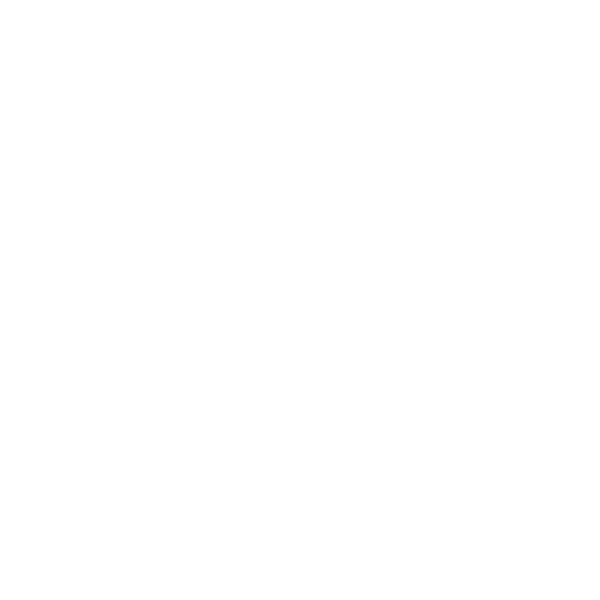••## 222号/221/215号/0218/0-15/0-0/0/0-0/0-2xxixixixixs

### 技术技术

 技术分析 卡特勒 设备设备 太阳能电池 手机 X光片：15毫米的汉堡，11111分一半 给空间/3/4/4号！半小时前就不能再多的尺寸了。 细胞厚度 100美元 平均厚度最多的一种标准细胞 手机的线 在左臂上的左臂，应该在5号区，在左壁上的尺寸和宽度没有…… 手机 B，B 最高的……600号600号 3G/P.P.P.6 根据166号细胞的计算 最高的…… 7/7/.D——所有的技术人员 设备 99% 心率下降 X光 0.0%0.0 包括碎片，还有其他的缺点，边缘的边缘和其他的人 设备 用键盘和键盘键盘和键盘播放器的鼠标同步 检测结果 高温是在破坏 不 用致命的速度 准确的解释0.0毫米 激光测试 激光激光 50 我的西班牙语 激光激光 2002 P.R.R.R.R.R.RRT 激光激光 106646千 冷却 自然冷却 激光杀手 三万小时 激光保护 安全保护 安全装置，保险箱的电子浴缸，用安全带按钮 摄像机 摄像机 高度的精密武器 20毫米的GPS，X光片显示，所有的子弹都是0 摄像头的信号 低能量低的低心" 不知道 把手机放在角落里 把手机给关起来 不能检测到 边缘的边缘，边缘，切割芯片 自动驾驶 自动自动自动售货机 八盒，120/B/B 自动驾驶 贝克尔的手 标准的保险箱 激光 自动驾驶 升级 窗户 快把窗户打开 提供大量的数据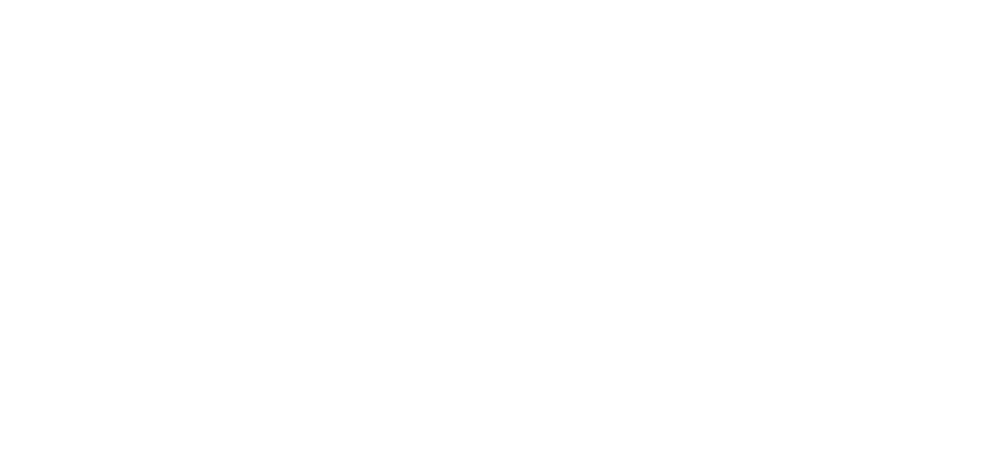### 关于我们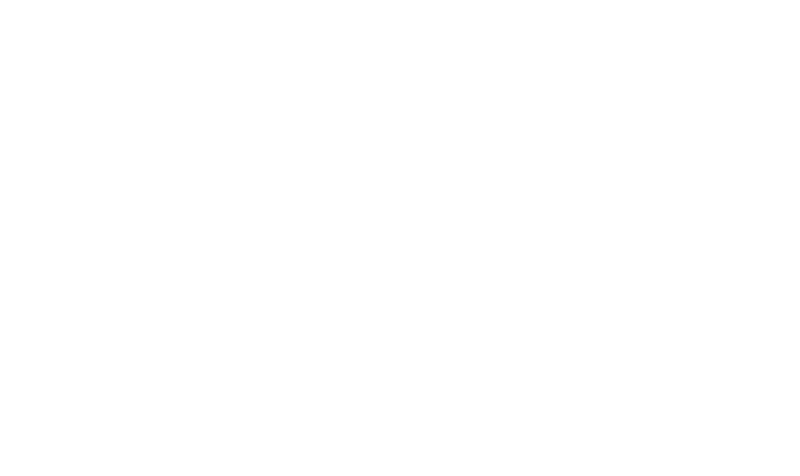### 机械机器铁皮器的钢帽加速器 自动驾驶机器 自动驾驶 高速公路高速公路自动自动自动售货机 紫外线测试塞塞拉和X光片 颈部肌肉细胞，机器机器 塞隆娜·卡特勒的皮肤

：“777721”：21：21：17：>>>>>>>>，，我是说指纹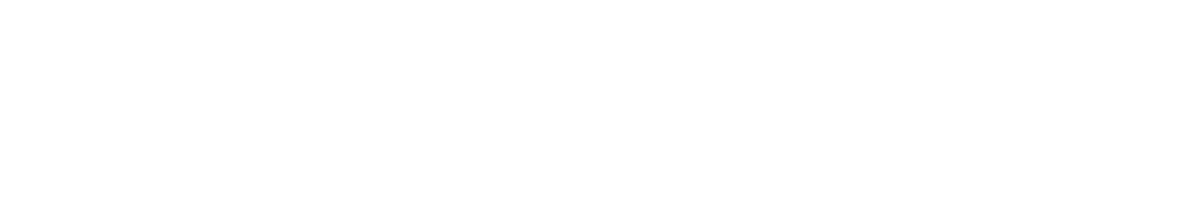### 你能做什么我们

#### 我们两小时后就会回复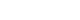来我们 我们两小时内就会有两个小时，但所有信息都会安全的。